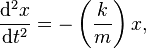Can someone please explain this simple harmonic motion equation for me?

Homework Statement

This is not a homework question, as we just started getting into SHM, and I want to be 100% clear what this equation means.

Can someone please all the parts, or have a site that really explains this formula?The Attempt at a Solution

Do you know what x is?
Do you know what is meant by dx/dt ?
Do you know what is meant by dv/dt ?

Do you know what x is?
Do you know what is meant by dx/dt ?
Do you know what is meant by dv/dt ?

No not really. I am looking for a clear explanation of what these all mean.

Thanks!

Is x the x = 0 equilibrium position?

Doc Al
Mentor
Is x the x = 0 equilibrium position?
Yes.

You can think of that equation as an application of Newton's 2nd law for a force obeying Hooke's law.

The second derivative of position wrt time is the same as acceleration, so all this equation is saying is ma = -kx, which means that F = -kx, which is Hooke's Law. To understand what this means you first need to know what x is defined to be. It usually means displacement from the equilibrium position of the spring. Also, "F" usually means the force exerted by the spring. If we ignore the "-k" for a second, we see that the Force is proportional to displacement, which means the more the displacement of the mass, the larger the Force exerted by the spring. The "k" is the constant of proportionality and the minus sign indicates that the direction of the force is opposite to that of the displacement (i.e if you pull the mass to the right, the spring tends to pull it back to the left, which makes sense intuitively).